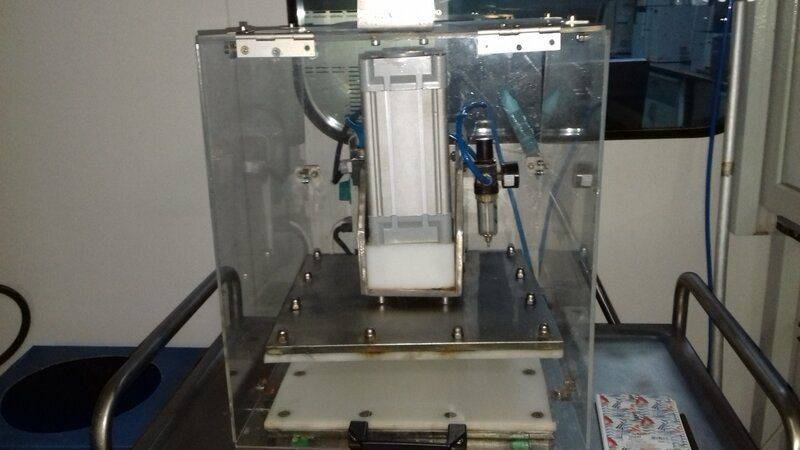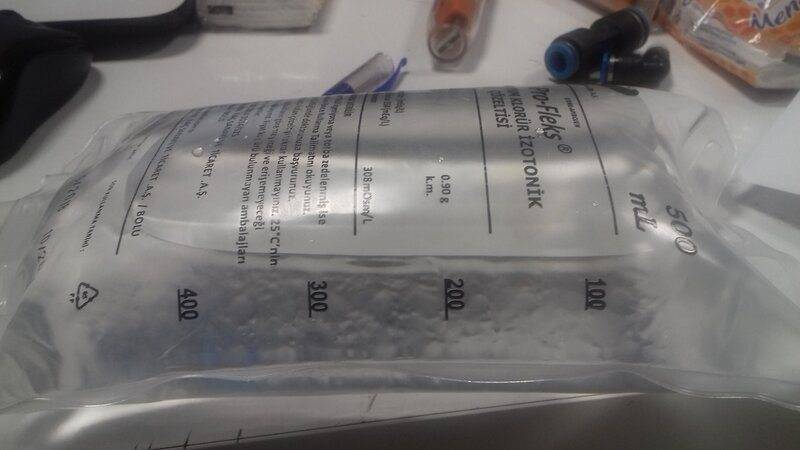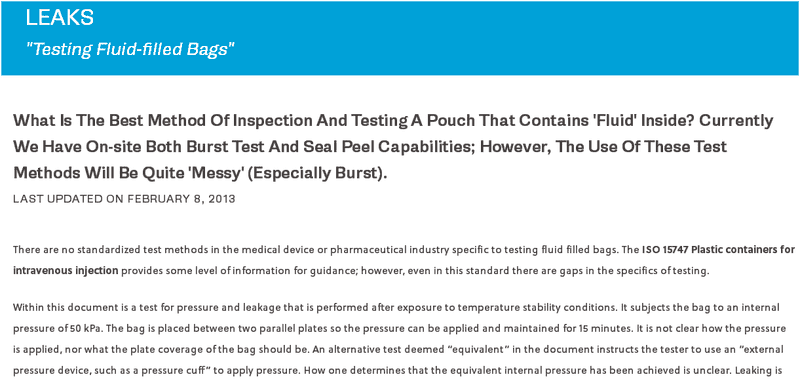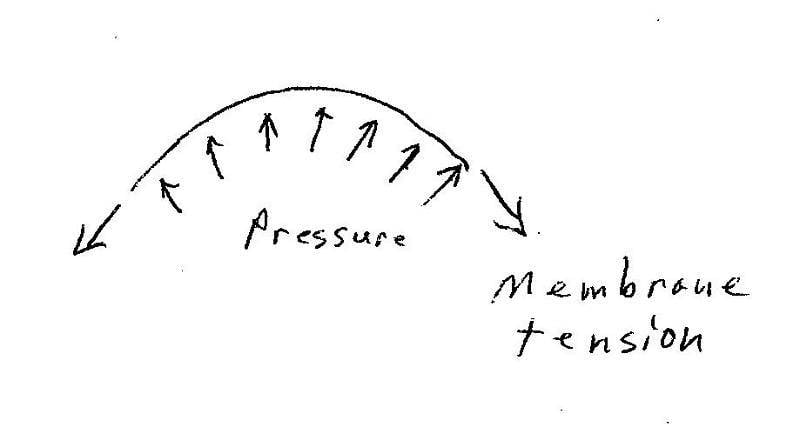# How to determine force based on pressure

• B
"leak under a certain pressure" is a bit more relevant, but "force acting on the bags" would not strike me as a useful quantity for quantifying leak...f

Hello
In a pharmaceutical lab,a certain pressure is applied to IV bags using the equipment shown below.We need to calculate the force acting on the bags based on the applied pressure.I know the formula is F=PxA.But i am not sure what the surface area is.Should i take the whole surface area of the empty bag or the part of the bag where the upper plate touches the bag or something else?The container is not completely filled with liquid.There is air above liquid.How does the compressed air affect the result?Welcome!
Compressible air acts like a spring, reducing its volume as the incompressible liquid is forced to find new space to occupy because the whole volume of the bag is reduced by the effect of the press.
In other words, the press is compressing the air.

So long as the plastic in the bag is flexible,, the pressure at the top of the bag is the Force supplied by the scale divided by the Area of the contact patch of that interface.
Note that the pressure at the bottom of the bag is very slightly higher because of the weight of the water.

Welcome to PF.

In a pharmaceutical lab,a certain pressure is applied to IV bags using the equipment shown below.We need to calculate the force acting on the bags based on the applied pressure.
Interesting. What is this test used for? Does this lab produce the filled IV bags, or do they just manufacture the empty bags but need to do this test on a sampling basis to check the quality/integrity of the bags?

Also, what is the purpose of calculating the force on the bag? Is it used to compare to the yield strength of the plastic bag material? Is there a published standard for performing this test? If there is, it probably lists the procedure for calculating the force on the plastic.

Note that because the IV bag is not a uniform shape (like a sphere), and because it has seams, the force distribution will not be uniform...

•Lnewqban
Is there a published standard for performing this test?
Hmm, with a littel Google searching, it looks like there are some standards, but not necessarily a universal standard for testing IV bags.https://www.sterilizationpackaging.org/faqs/questions/82

•Lnewqban

Note that because the IV bag is not a uniform shape (like a sphere), and because it has seams, the force distribution will not be uniform...
The pressure will be uniform, what is not uniform is the surface tension (force/length) in the poly which is subject to Young-Laplace law involving the local curvature.

•berkeman
Thank you for the replies.
I have another thought. I could find the force acting on the plate by the air cylinder multiplying air pressure by the piston area.Is that right?How much of this force can be transferred to bags.?Should I add the weight of the plate?

Thank you for the replies.
I have another thought. I could find the force acting on the plate by the air cylinder multiplying air pressure by the piston area.Is that right?How much of this force can be transferred to bags.?Should I add the weight of the plate?
It would be helpful if you could answer my questions in Post #4 first. Thank you.

What is this test used for? Does this lab produce the filled IV bags, or do they just manufacture the empty bags but need to do this test on a sampling basis to check the quality/integrity of the bags?
This lab is the part of a pharmaceutical manufacturing company in Turkey.The lab performs some tests on the bags of IV solution produced at the same building.
Also, what is the purpose of calculating the force on the bag? Is it used to compare to the yield strength of the plastic bag material? Is there a published standard for performing this test? If there is, it probably lists the procedure for calculating the force on the plastic.
Actually,I don't have the details.I don't work in the lab.The test is done to find out whether bags of IV solution leak under a certain pressure.The lab manager has asked me to find a way to determine the force acting on the bags.I didn't ask him the reason.

Over what part of the bag is the external force applied, and in what direction?

Actually,I don't have the details.I don't work in the lab.The test is done to find out whether bags of IV solution leak under a certain pressure.The lab manager has asked me to find a way to determine the force acting on the bags.I didn't ask him the reason.
"force acting on the bags" would not strike me as a useful quantity for quantifying leak thresholds.

This is sort of like using the contact patch of a tires and the weight of the car to figure the tire pressure...it has some merit as a worst case test, I think. If the bags are similar in shape and you push on them in the same geometry it might work. It certainly depends upon the requirements of the test!

If the bag is thin and flexible, such that bending moments in the bag are negligible, then there are two different conditions:

Case 1) The bag is curved. The free body diagram below shows the tensile forces in the bag and the internal pressure. Since the sum of forces equal zero in all directions, the tensile force per unit length of bag can be easily calculated.Case 2: The bag is flat, such as when in contact with a flat surface. In this case, the tensile stress in the bag is undetermined, and the internal pressure is equal to the external pressure. Since the external surface is horizontal, and the internal pressure is from a fluid, the pressure is equal across the entire bag area in contact with a single flat surface. That pressure is then the total applied force divided by the actual contact area.

A car tire is thick enough that there will be some contact pressure variation due to bending stresses in the tire. Those bending stresses are usually fairly small, and can be neglected as a first order approximation.

Absolutely
The pressure will be uniform, what is not uniform is the surface tension (force/length) in the poly which is subject to Young-Laplace law involving the local curvature

Absolutely
Careful, the newbie you are quoting can be unreliable at times...•hutchphd